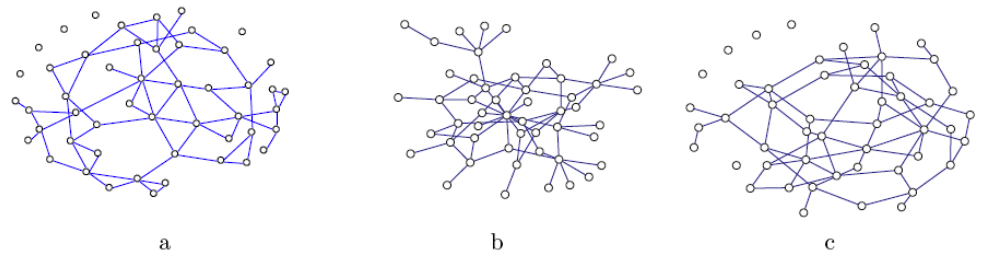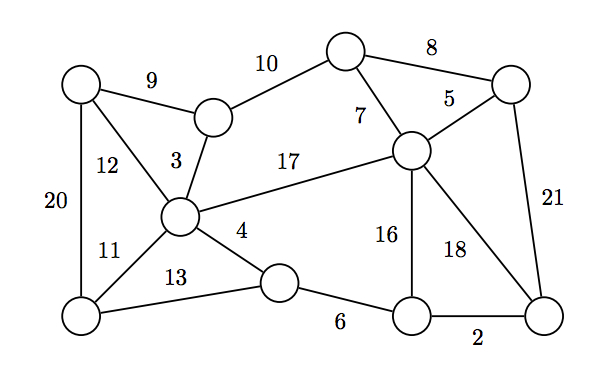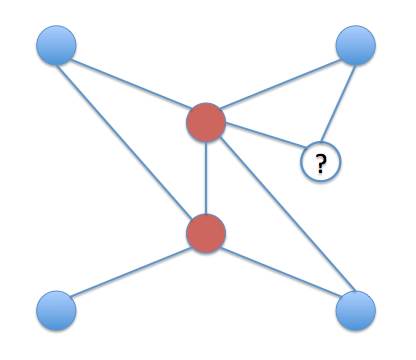### Network Analysis - Assignment 3

Due March 20, 2013 by 11:59pm

You should submit a write-up of your assignment both in pdf form and in hardcopy.  The pdf is due by electronic submission before the due date and time.  The electronic submission timestamp is what counts for determining whether your assignment was submitted on-time.  You may turn the hardcopy printout in up to three days later, but it must be identical to the electronic submission.  You will also submit your code electronically.

To submit your assignment, place a copy of your write-up (pdf preferred) and implementation into a directory called cs380hw3_lastname (substituting in your last name), then tar and gz this directory.  Name the file cs380hw3_lastname.tar.gz.  Submit it to me by attaching it to an e-mail with the subject of "CS380 Assignment 3 Lastname".  Make sure you give me a hard-copy of your write-up for the assignment.

1.)  [14 points]  Small World Networks

a.)  [6 pts] Which of the following graphs has the highest clustering coefﬁcient? (You don’t need to actually compute it!)  Justify your answer.b.) [8 pts] We introduced two variants of the Watts/Strogatz small world model (edge rewiring and edge addition).  Which model leads to the larger clustering coefﬁcient? The larger average path length?  Justify your answers.

2.)  [20 points]  Minimum Spanning Trees

a.)  [5 pts] Using one of the algorithms discussed in class, find a minimum spanning tree for the network below.  Describe the intuition behind that algorithm and give the MST.b.)  [10 pts] "If G is an arbitrary connected, undirected graph with a non-negative weight on every edge, and e* is the edge with the lowest weight in G, then there is a minimum spanning tree T of G that contains the edge e*."  Is this statement true or false?  Justify your answer.

c.)  [5 pts]  Does squaring the weights of a non-negative weighted graph change the minimum spanning tree?  Why or why not?

3.)  [16 points]  Learning in Networks

Given the following network, construct a naive Bayes classifier to predict the color of a vertex based on its degree and unnormalized betweenness centrality. Discretize each of these features into either two or three bins, as appropriate.  Start by specifying a table of the training data for the classifier.  Then, specify the naive Bayes classifier by listing all prior and conditional probabilities necessary for the model and their corresponding values.  (If you can derive some of the parameters from others, than these parameters can be omitted.)What color would the model predict for the uncolored node (?) in the network?  Show your computations!

4.)  [50 points]  Small World Networks

Write a small world network generator that supports both Watts/Strogatz small world variants that we discussed in class.  Conduct a small scientific experiment of how the network properties change as you vary the number of vertices N, the probability p of either rewiring or adding edges, and the number of nearest neighbors k for both small world model variants.  Show how the following change:
• average localized clustering coefficient
• average path length (for this one it will probably be easiest to implement Floyd-Warshall)
• average degree centrality
• centralization

by plotting the ratio of their values for the small world network vs the original lattice (e.g., C(p) / C(0) and l(p) / l(0), as shown on the slides).  Your answer should include a number of 2D plots (3D is okay as well, if you'd like a challenge) and a written explanation explaining the results and comparing them for both small world model variants.